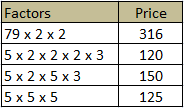## Thursday, August 11, 2011

### The \$7.11 Problem

This problem comes from Jim Wilson's math site from the University of Georgia (http://jwilson.coe.uga.edu/), and it is very interesting to analyze.
* Problem from Professor Doug Brumbaugh, University of Central Florida.

A guy walks into a 7-11 store and selects four items to buy. The clerk at the counter informs the gentleman that the total cost of the four items is \$7.11. He was completely surprised that the cost was the same as the name of the store. The clerk informed the man that he simply multiplied the cost of each item and arrived at the total. The customer calmly informed the clerk that the items should be added and not multiplied. The clerk then added the items together and informed the customer that the total was still exactly \$7.11.

What are the exact costs of each item?
So where should I begin to start solving the problem?

In looking for the 4 numbers that add and multiply to \$7.11 I find that it is easy to come up with four numbers whose sum is correct, and it is much more difficult to think about numbers whose product is correct.

Thus, do find numbers that will multiply my first instinct is to factor the number. When I factored 7.11 I found that 3, 3, and 0.79 divide into it evenly. But 0.79 + 3.00 + 3.00 + 1.00 = 7.79. So I will have to manipulate the factors to accommodate for the decimals.

Decimals are nasty to work with, so I will convert everything into pennies. My sum is then multiplied by 100 to give me 711, and the product is multiplied by 100^4 to give me 711,000,000. This can be seen in the equations below:
100a + 100b + 100c + 100d = 100 (a + b + c + d) = 100 (7.11) = 711
100a * 100b * 100c * 100d = (100^4)(a * b * c * d) = (100^4)(7.11) = 711,000,000
Now when I factor 711,000,000 I can see that I am left with 79, 3, 3, 2, 2, 2, 2, 2, 2, 5, 5, 5, 5, 5, and 5. So now it's a matter of rearranging these factors so that their four products sum to 711.I begin with 79 since it is the largest and will yield the fewest combinations. I can determine that one of my prices is a multiple of 79 cents as seen in the chart. I did not include 79 * 3 * 3 = 7.11 because then the other prices would be zero and the multiplication would fail.

From here forward the method to solve is based on combinatorics (combinations). To narrow my permutations, I can first use some logic.

At least one of the remaining numbers ends in something other than a 0 because none of the above end in 1. Thus, in order for the ones digit of our sum to be a 1, we need the digits to add to 1, 11, 21, 31. This means that at least one number will not contain a factor of 5.

For numbers 79, 158, 237, 474, and 632, this means that the six 5's would be split among two prices which would total at least 125 cents each. So I can rule out 474 and 632 as possible values because 125 + 125 = 250 and 474 +250 > 711 as well as 632 + 250 > 711.

It is at this point that I notice a pattern that gives me a hunch. My guess is that the value will be either 3.16 or 3.95. Why? Because these numbers allow for the six factors of 5 to be spread among three prices instead of two.I begin with 3.16 so that I can examine the prices who all have factors of 5. So for my bases I have 316; 5*2; 5*2; and 5. This leaves me with the remaining factors of 3, 3, 2, 2, 5, 5, and 5. With addition limiting the maximum and minimum sizes of the prices, I can quickly come to find that the four prices are \$3.16, \$1.20, \$1.50, and \$1.25.

Share some of your strategies (successful or not) in the comments below. Are there any other solutions to be found? How about factors that aren't constrained to integer values for pennies?